# Organic Synthesis – list of solved problems

Go to the page with the solutions to the problems.

## Organic Synthesis – list of problems

The following list of Organic Synthesis problems deals with the three aspects described below:

• Reactivity of molecules with more than one functional group.
• Synthetic sequences involving the chemistry of different functional groups.
• Preparation of molecules from fragments with a smaller number of carbon atoms.

### Problem 1)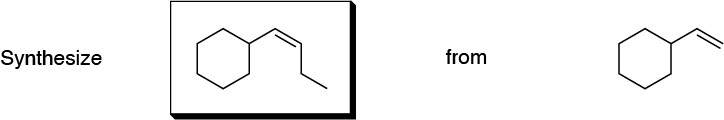### Problem 2)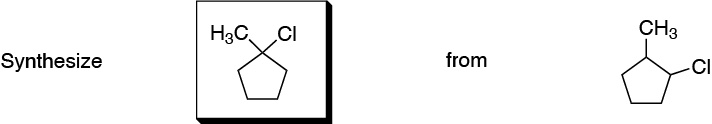### Problem 3)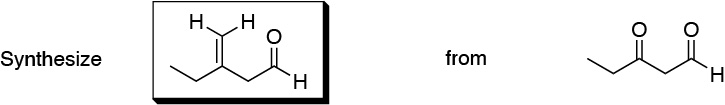### Problem 4)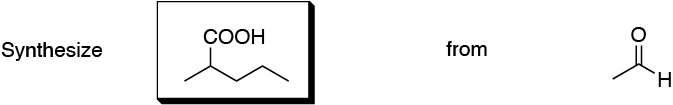### Problem 5)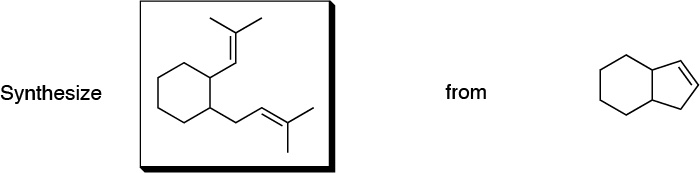### Problem 6)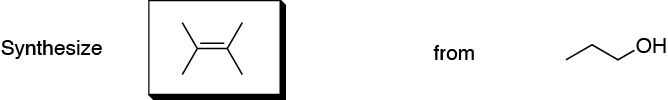### Problem 7)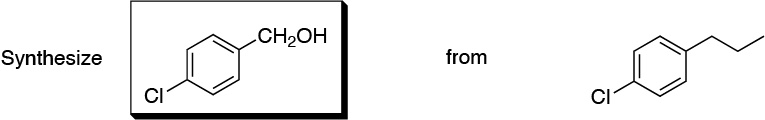### Problem 8)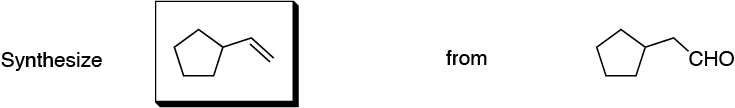### Problem 9)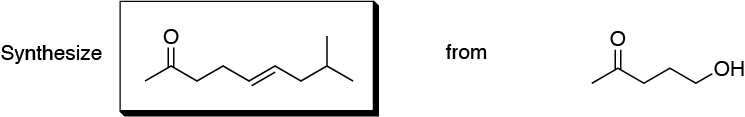### Problem 10)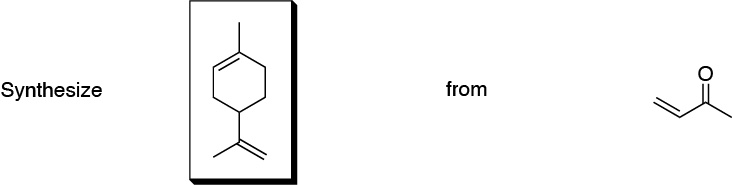### Problem 11)

From acetylene and as many reagents as you think necessary, prepare the Z and E isomers of 6-methylhept-3-ene.

### Problem 12)

Obtain 1-phenylpropan-2-one from (bromomethyl)benzene.

### Problem 13)

Design a procedure to obtain 2-phenylacetamide from bromobenzene.

### Problem 14)

Suggest a procedure to perform the following transformations: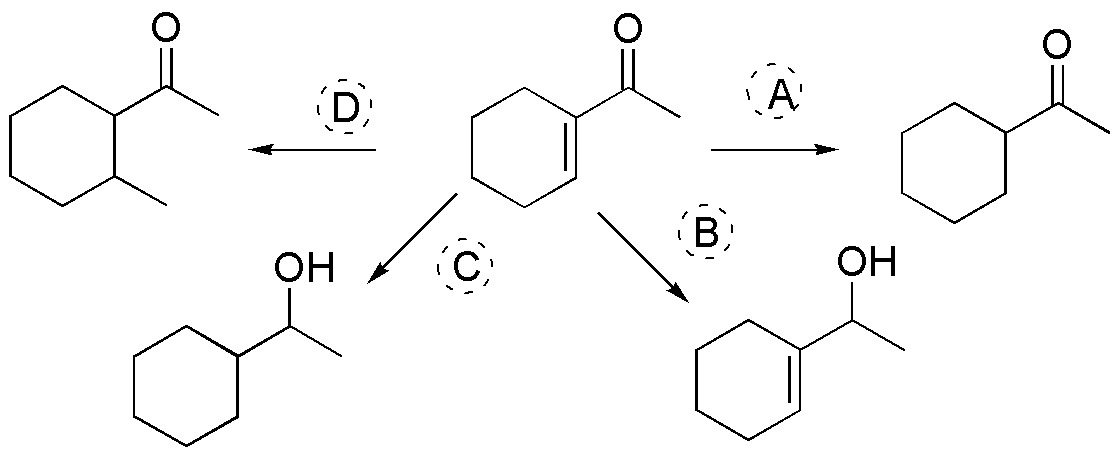### Problem 15)

Pentyl bromide is reacted with magnesium in ether to give compound A. A reacts with ethylene oxide followed by aqueous acid to give B. When B is oxidized with PPC in CH2Cl2 it gives C, which reacts with phenylmagnesium bromide to give D, after treating with aqueous hydrochloric acid. Determine the structures of compounds A, B, C and D, based on the sequence of reactions described.

### Problem 16)

Describe a procedure to obtain 6-methyltetrahydro-2H-pyran-2-one (δ-caprolactone) from 4-bromobutanal.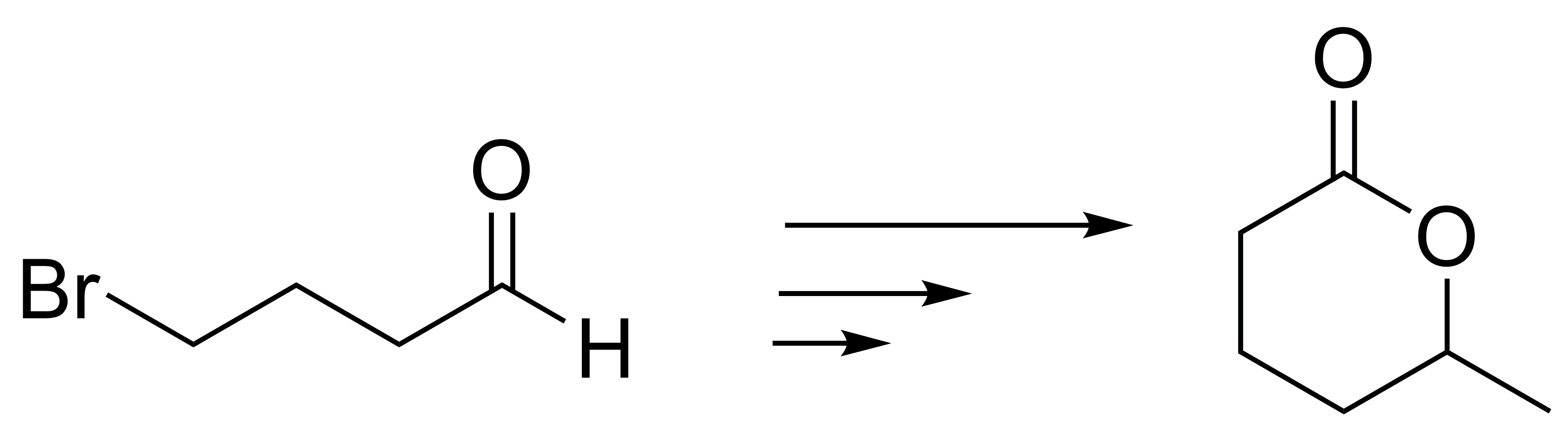### Problem 17)

Employing cyclohex-2-enone as starting material prepare 4-oxocyclohex-2-enocarboxylic acid.

### Problem 18)

Ethyl p-aminobenzoate is commercially known as benzocaine. It is widely used in medicine as a local anesthetic. Design a procedure to obtain such a product from benzene.

### Problem 19)

Design a procedure for the synthesis of methyl pentanoate from 1-bromobutane.

### Problem 20)

Using as starting products or carbon source compounds (I-V), design a synthetic procedure for the preparation of a) and b).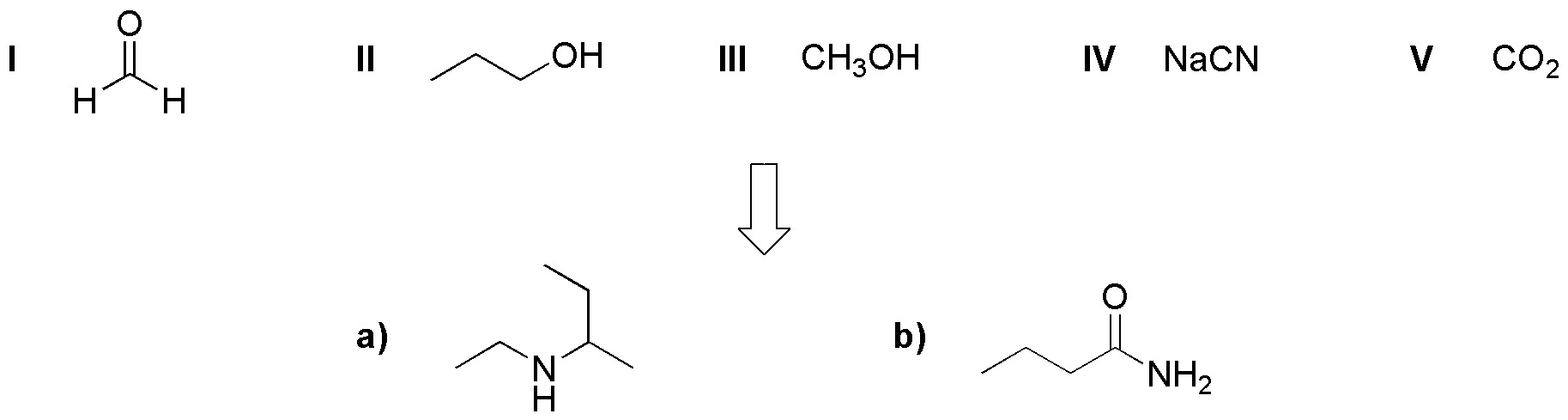### Problem 21)

Starting from ethanol, as the only carbon compound, describe a synthesis for the following molecules:### Problem 22)

Deduce in a reasoned way, which compounds from the following list will be obtained more easily by a malonic synthesis.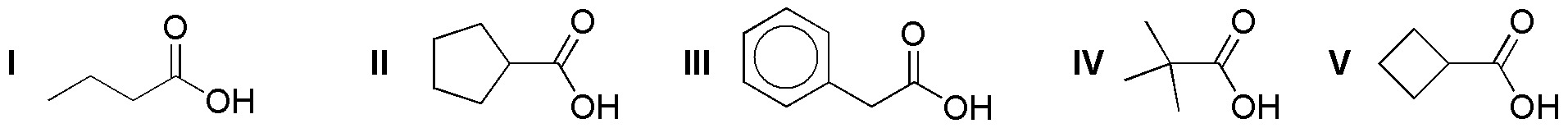### Problem 23)

An alkene of molecular formula C6H12 gives by ozonolysis propanal as the only product. Treatment with a peracid leads to an optically active compound. Propose the structure of such an alkene.

### Problem 24)

Complete the following table, taking into account the stereochemistry of the compounds obtained (A-X).

Br2 / Cl4C H2O2 / OsO4 (cat.) Ozonolysis KMnO4 (hot) Peracids HBr / peroxides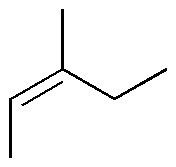A B C D E F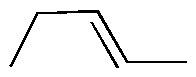G H I J K L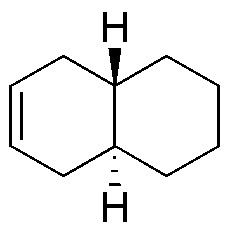M N O P Q R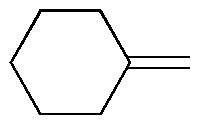S T U V W X

### Problem 25)

Complete the following table (A-I) of reactivity of alcohols.

PCC /CH2Cl2 CrO3 / H3O+ KMnO4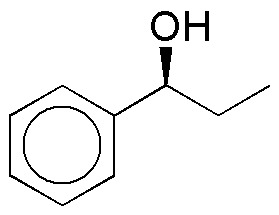A B C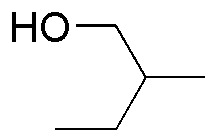D E F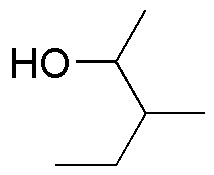G H I

### Problem 26)

Complete the following table (A-P):

LiAlH4 NaBH4 B2H6 DIBAH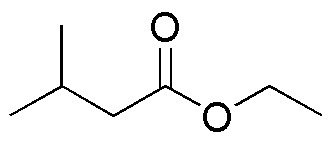A B C D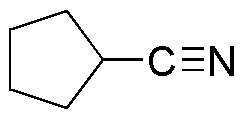E F G H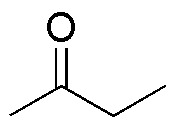I J K L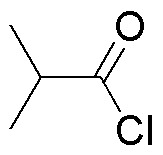M N O P

### Problem 27)

Complete the following scheme (A-K):

Design a procedure to prepare propane-1,1,1,2,3-tetracarboxylic acid from: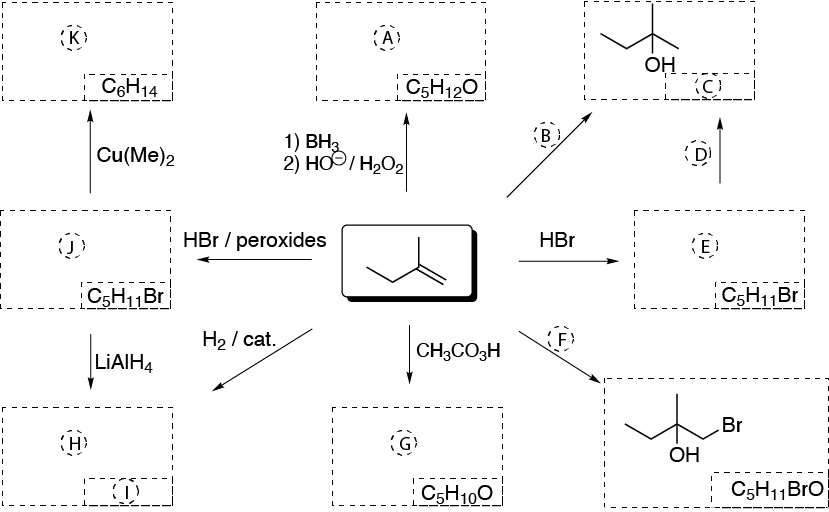### Problem 28)

Indicate the reagents (A-M) needed to perform the following transformations: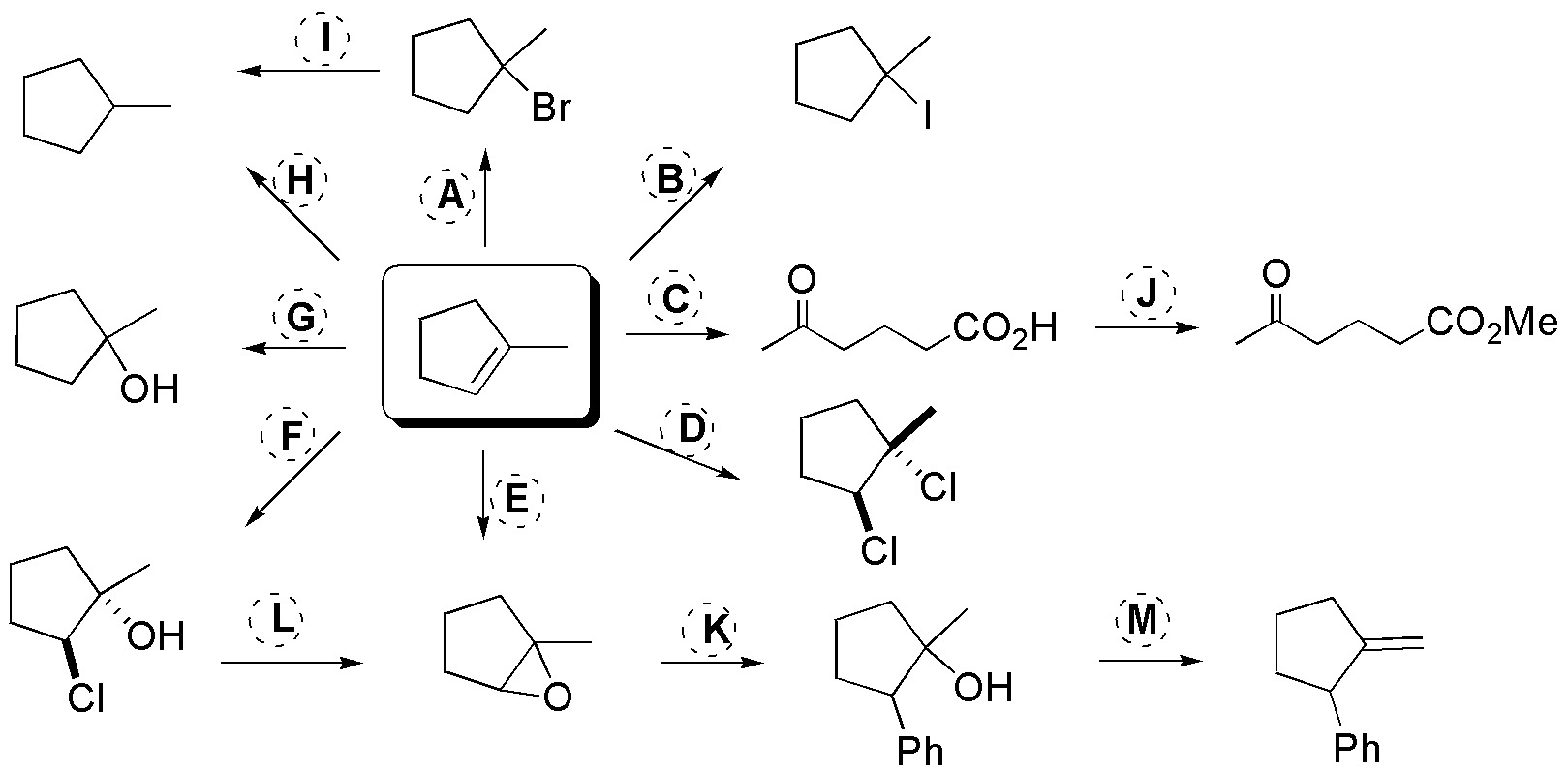### Problem 29)

Deduce the structures of the compounds A-F.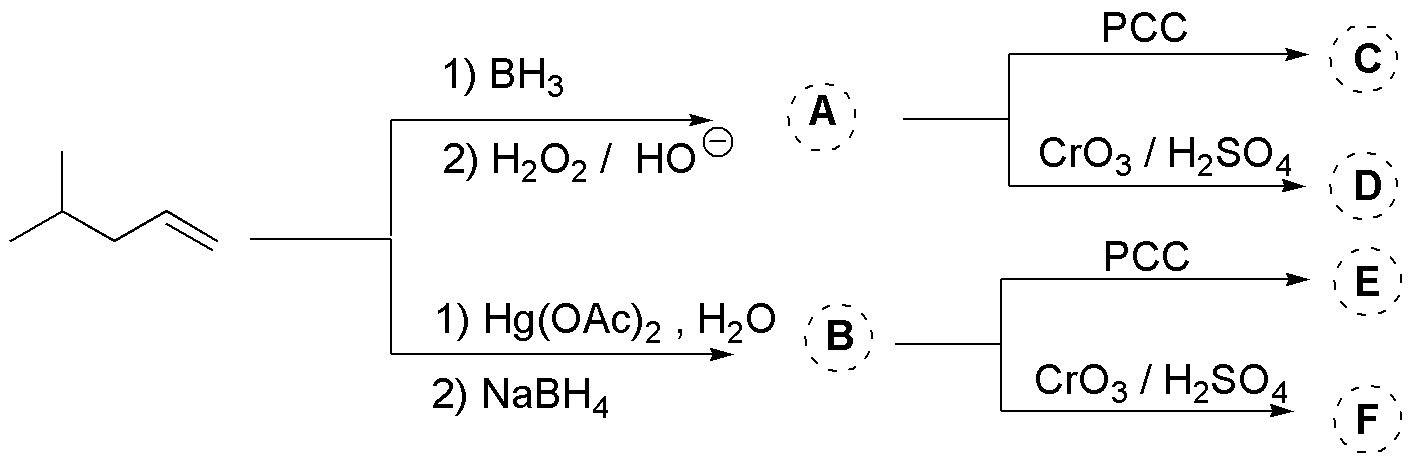### Problem 30)

Complete the scheme (A-G):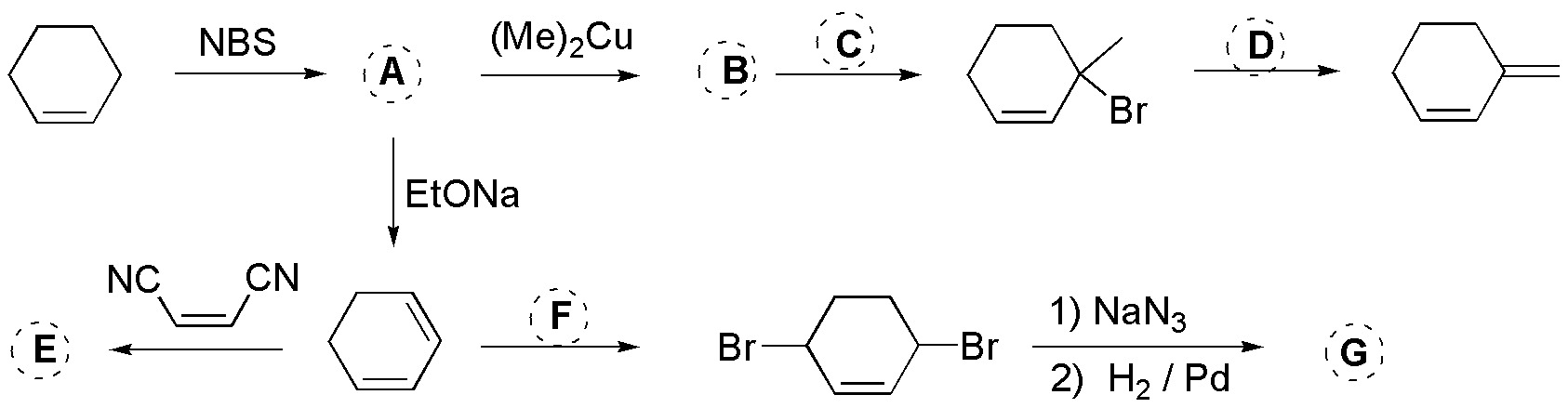### Problem 31)

Deduce the structures of the compounds (A-G) shown in the scheme, from the reactions that take place.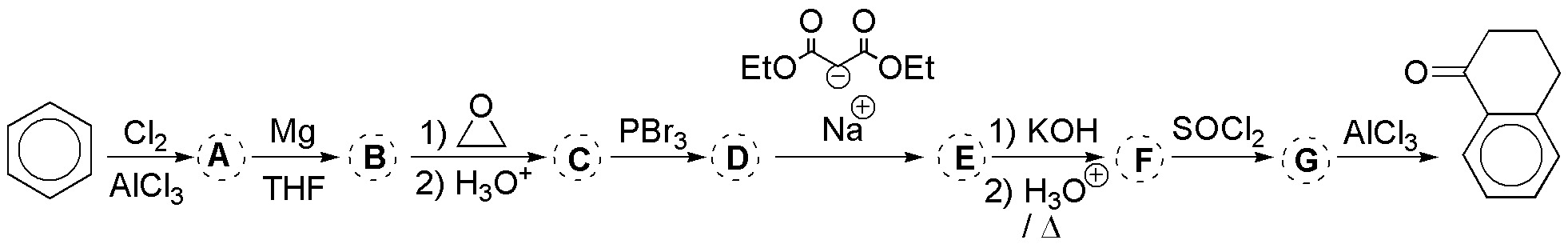### Problem 32)

Propose a procedure to perform the following transformations (a-d):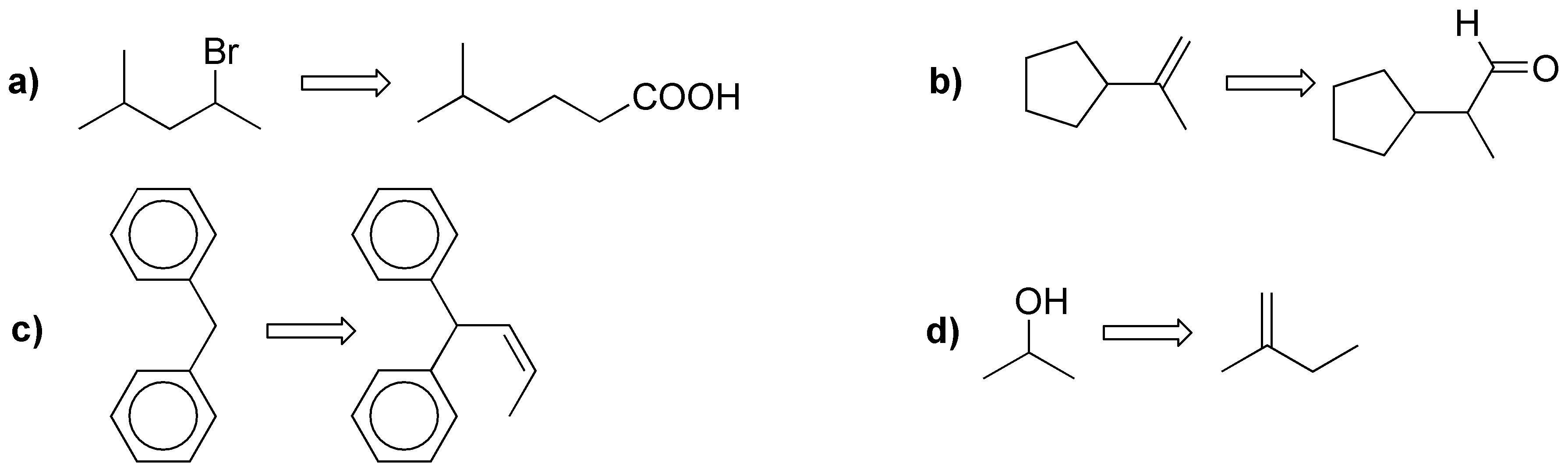### Problem 33)

Complete the scheme, describing the reactants A-G.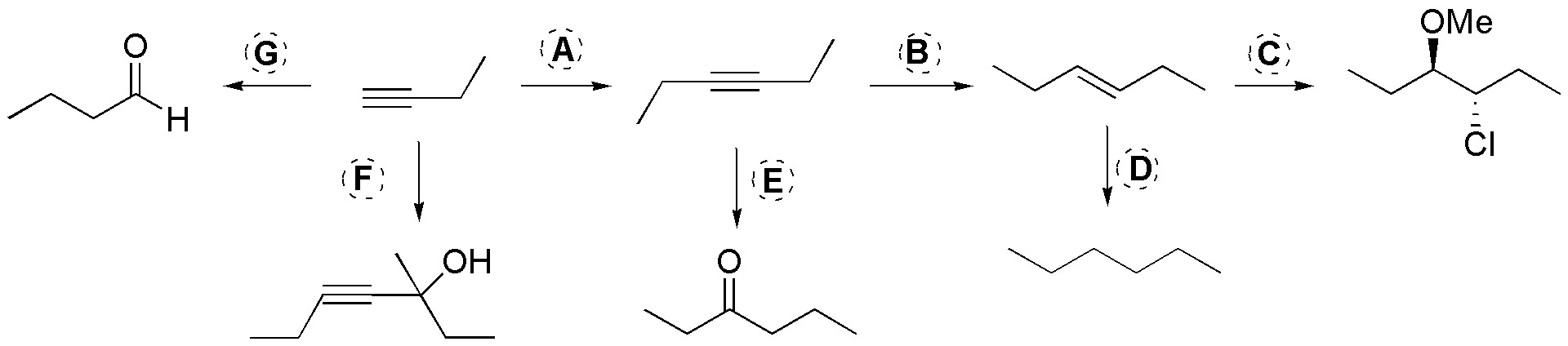### Problem 34)

Complete the following scheme (A-E), indicating the reagents needed for this purpose, and keeping in mind that transformations A and D require two steps.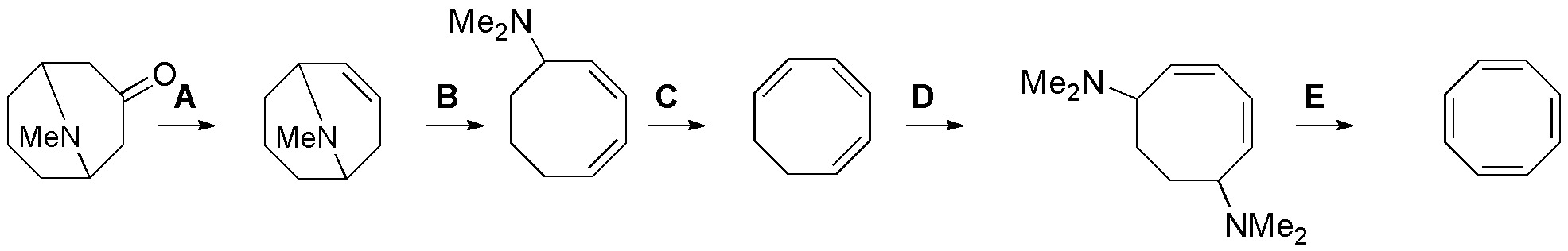### Problem 35)

Design a procedure to perform the following synthesis: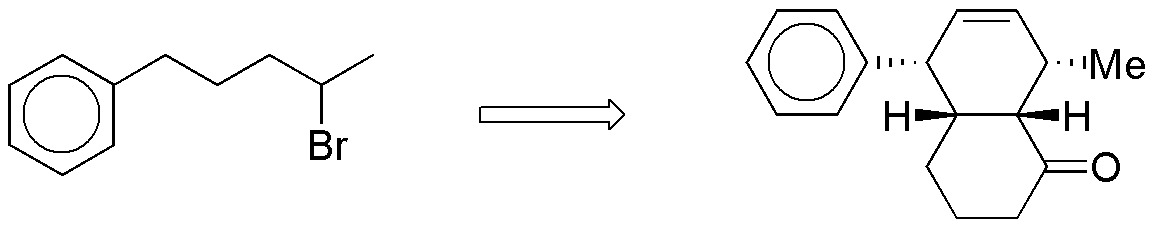### Problem 36)

Complete (A-D) the synthesis of 5-ethyl-6-methylhepta-1,6-diol from allyl bromide, according to the following scheme: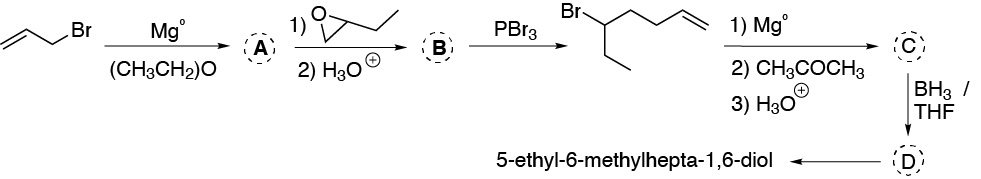### Problem 37)

Describe the reactions necessary to transform the reagent methyl 6-oxoheptanoate into the product 6-oxoheptyl benzoate.

### Problem 38)

When acetic acid is treated with bromine in the presence of phosphorus, compound A is obtained. Reaction of A with NaCN / DMSO leads to the formation of B. If B is reacted with an aqueous mineral acid in the presence of ethanol C (C7H12O4) is obtained. If C is treated with sodium ethoxide in ethanol, followed by benzyl bromide leads to the formation of D (C14H18O4). Hot acid hydrolysis of D leads to E (C9H10O2). Deduce the structures of A, B, C, D and E.

### Problem 39)

A compound A of molecular formula C7H14O can be obtained from B (C6H10O) or from C (C7H12) interchangeably. When it is obtained from B, lithium dimethylcuprate is needed, whereas if it is prepared from C it is necessary to use diborane, followed by H2O2 in basic medium. Determine the structures of A, B and C, knowing that B is synthesized from cyclohexene and peracetic acid.

### Problem 40)

Bromobenzene reacts with magnesium in THF to give compound A. A is transformed by bubbling CO2 and treating with an acid, to give B (C7H6O2). Treatment of B with methanol in acid medium leads to C (C8H8O2). When C is reacted with 2 mol of A and subsequent acidification, D (C19H16O) is obtained. Deduce the structures A, B, C and D based on the reactions described.

### Problem 41)

Design a synthetic route to perform the following transformation: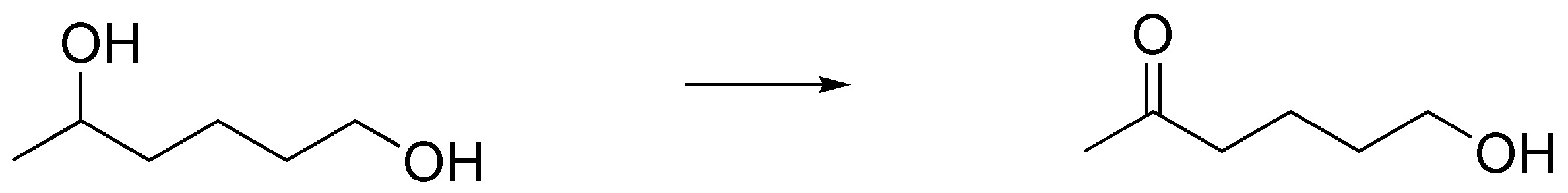### Problem 42)

Cyclopentene when treated with a mineral acid leads to the formation of a compound A which when the corresponding calculation is carried out, presents an unsaturation. When A is treated with potassium dichromate in an acidic medium B is obtained. The reaction of B with m-chloroperbenzoic acid leads to the formation of C. Deduce the structures of compounds A-C.

### Problem 43)

Design a feasible procedure for the preparation of the following compounds (a-d) by malonic or acetylacetic and related syntheses.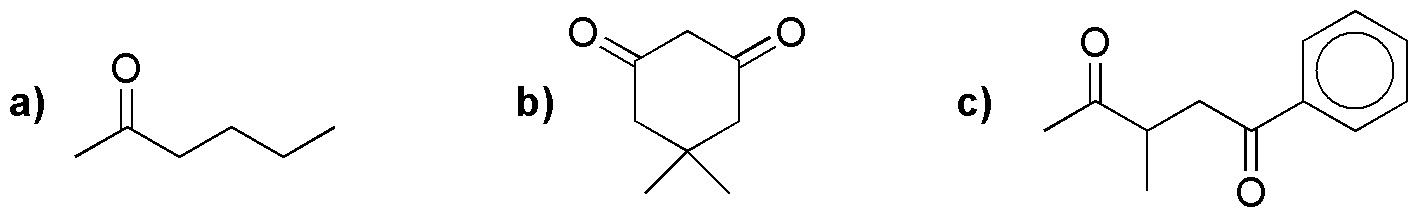### Problem 44)

The molecule known as disparlura is a pheromone of a moth species, the synthesis of which is partially described in the following scheme. Deduce the structure of the compound.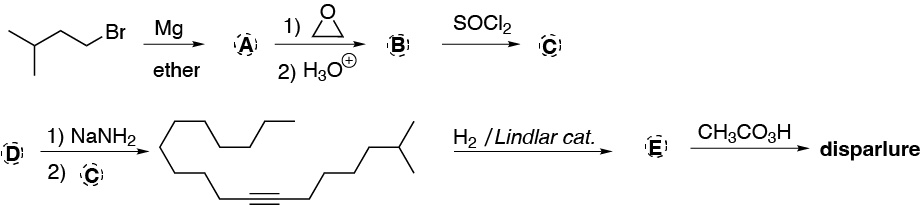### Problem 45)

From cyclohexanone, prepare the following compounds (a-d):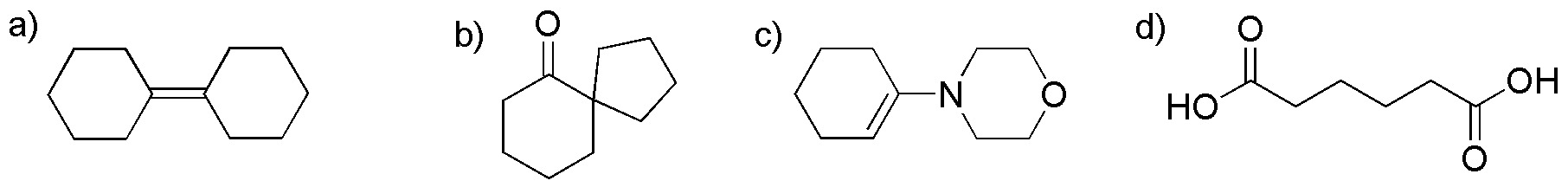### Problem 46)

Design a procedure for the preparation of the following compound from benzenonitrile.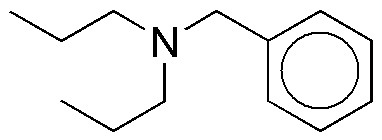### Problem 47)

Starting from cyclopentene, prepare compounds (a) and (b).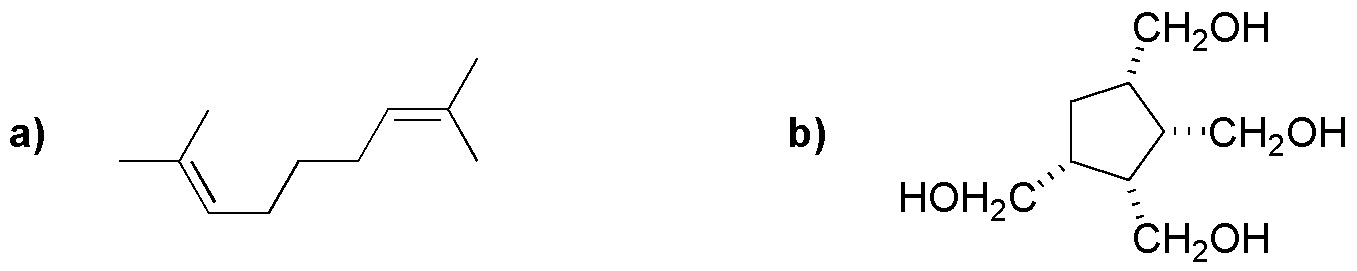### Problem 48)

Complete the scheme, describing the reagents A-F.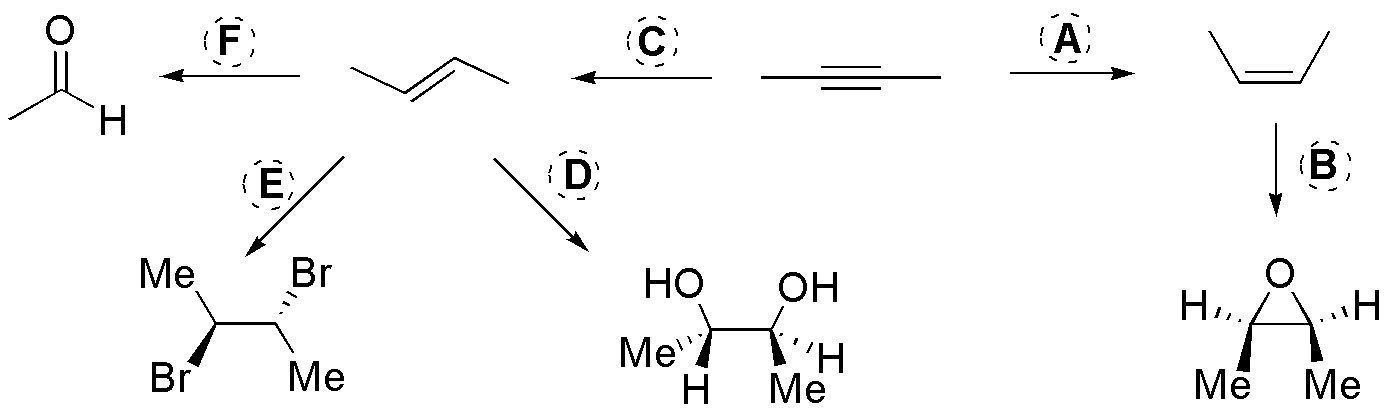Shares